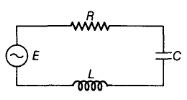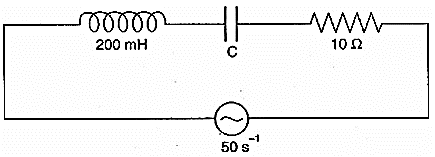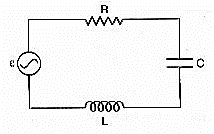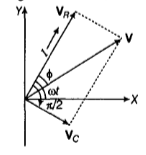# Alternating Current Class 12 Physics Extra Questions## myCBSEguide App

Complete Guide for CBSE Students

NCERT Solutions, NCERT Exemplars, Revison Notes, Free Videos, CBSE Papers, MCQ Tests & more.

Alternating Current Class 12 Physics Extra Questions. We know Physics is tough subject within the consortium of science subjects physics is an important subject. But if you want to make career in these fields like IT Consultant, Lab Technician, Laser Engineer, Optical Engineer etc. You need to have strong fundamentals in physics to crack the exam. myCBSEguide has just released Chapter Wise Question Answers for class 12 Physics. There chapter wise Extra Questions with complete solutions are available for download in myCBSEguide website and mobile app. These Questions with solution are prepared by our team of expert teachers who are teaching grade in CBSE schools for years. There are around 4-5 set of solved Physics Extra Questions from each and every chapter. The students will not miss any concept in these Chapter wise question that are specially designed to tackle Board Exam. We have taken care of every single concept given in CBSE Class 12 Physics syllabus and questions are framed as per the latest marking scheme and blue print issued by CBSE for class 12.

Class 12 Physics Extra Questions

## Physics Class 12 Chapter 7 Latest Exam Questions

Class – 12 Physics (Alternating Current)

1. A 200 ohm resistor is connected in series with a {tex}5\mu F{/tex} capacitor. The voltage across the resistor is VR = (1.20 V) cos(2500 rad/s)t.Capacitive reactance is

1. 70 {tex}\Omega {/tex}
2. 80 {tex}\Omega {/tex}
3. 60 {tex}\Omega {/tex}
4. 90 {tex}\Omega {/tex}
2. The current in a series LCR circuit will be maximum, then {tex}\omega {/tex} is:

1. as large as possible
2. {tex}\sqrt {LC} {/tex}
3. {tex}\sqrt {LCR} {/tex}
4. equal to natural frequency of LCR system
3. A series circuit consists of an ac source of variable frequency, a 115.0 {tex}\Omega {/tex} resistor, a 1.25 {tex}\mu {\rm{F}}{/tex} capacitor, and a 4.50-mH inductor. Impedance of this circuit when the angular frequency of the ac source is adjusted to twice the resonant angular frequency is

1. 146.0 {tex}\Omega {/tex}
2. 176.0 {tex}\Omega {/tex}
3. 166.0 {tex}\Omega {/tex}
4. 156.0 {tex}\Omega {/tex}
4. For high frequency capacitor offers:

1. Less resistance
2. More resistance
3. None of these
4. Zero resistance
5. Effective voltage Vrms is related to peak voltage Vo by

1. Vrms = 0.707 Vo
2. Vrms = 0.787Vo
3. Vrms = 0.9 Vo
4. Vrms = 0.5 Vo
6. An AC current, I = I0 sin {tex}\omega t{/tex} produces certain heat H in a resistor R over a time {tex}T\, = 2\pi /\omega {/tex}. Write the value of the DC current that would produce the same heat in the same resistor in the same time.
7. Define the term rms value of the current. How is it related to the peak value?

8. Define the term wattless current.

9. The figure shows a series L-C-R circuit connected to a variable frequency 250 V source with L = 50 mH, C = 80{tex}\mu {/tex}F and R = 40{tex}\Omega {/tex} .1. the source frequency which drives the circuit in resonance.
2. the quality factor (Q) of the circuit.
10. The number of turns in secondary coil of a transformer is 100 times the number of turns in the primary coil. What is the transformation ratio?

11. In the following circuit, calculate,

1. the capacitance ‘c’ of the capacitor if the power factor of the circuit is unity, and
2. also calculate the Q-factor of the circuit.12. A source of AC voltage V = V0 sin {tex}\omega t{/tex} is connected to a series combination of a resistor ‘R’ and a capacitor ‘C’. Draw the phasor diagram and use it to obtain the expression for

1. impedance of the circuit and
2. phase angle.
1. For a given AC, i = im sin {tex}\omega t{/tex} , show that the average power dissipated in a resistor R over a complete cycle is {tex}\frac { 1 } { 2 } i _ { m } ^ { 2 } R{/tex}.
2. A light bulb is rated at 100 W for a 220V AC supply. Calculate the resistance of the bulb.
13. An LC circuit contains a 20 mH inductor and a {tex}50\mu F{/tex} capacitor with an initial charge of 10 mC. The resistance of the circuit is negligible. Let the instant the circuit is closed be t = 0.

1. What is the total energy stored initially? Is it conserved during LC oscillations?
2. What is the natural frequency of the circuit?
3. At what time is the energy stored
1. completely electrical (i.e. stored in the capacitor)?
2. completely magnetic (i.e. stored in the inductor)?
4. At what times is the total energy shared equally between the inductor and the capacitor?
5. If a resistor is inserted in the circuit, how much energy is eventually dissipated as heat?
14. A series of LCR circuit connected to a variable frequency 230 V source, L = 5.0 H, {tex}C = 80\mu F{/tex}, {tex}R = 40\Omega {/tex}1. Determine the source frequency which drives the circuit in resonance.
2. Obtain the impedance of the circuit and the amplitude of current at the resonating frequency.
3. Determine the rms potential drops across the three elements of the circuit. Show that the potential drop across the LC combination is zero at the resonating frequency.

Class – 12 Physics (Alternating Current)

1. 80{tex}\Omega {/tex}
Explanation: VR = (1.20 V) cos(2500 rad/s)t
{tex}C = 5\mu F = 5 \times {10^{ – 6}}F{/tex}
Capacitive reactance
{tex}{X_C} = {1 \over {\omega C}} = {1 \over {2500 \times 5 \times {{10}^{ – 6}}}} = 80\Omega {/tex}
1. equal to natural frequency of LCR system
Explanation: for maximum current in LCR series circuit impedance Z will be minimum
{tex}i = \frac{E}{Z}{/tex}
{tex}Z = \sqrt {{R^2} + {{({X_L} – {X_C})}^2}} {/tex}
impedance Z will be minimum when {tex}{X_L} = {X_C}{/tex}
hence
{tex}\omega L = \frac{1}{{\omega C}}{/tex}
{tex}\omega = \frac{1}{{\sqrt {LC} }}{/tex}
this is equal to natural frequency of LCR system
1. 146.0
Explanation: {tex}R = 115\Omega {/tex}
{tex}C = 1.25\mu F = 1.25 \times {10^{ – 6}}F{/tex}
{tex}L = 4.5mH = 4.5 \times {10^{ – 3}}H{/tex}
resonant angular frequency
{tex}{\omega _0} = {1 \over {\sqrt {LC} }} = {1 \over {\sqrt {4.5 \times {{10}^{ – 3}} \times 1.25 \times {{10}^{ – 6}}} }} = {1 \over {7.5 \times {{10}^{ – 5}}}}{/tex}
given that the angular frequency of the ac source {tex}\omega = 2{\omega _0} = {2 \over {7.5 \times {{10}^{ – 5}}}} = 26666.6rad/s{/tex}
impedance
{tex}Z = \sqrt {{R^2} + {{\left( {\omega L – \frac{1}{{\omega C}}} \right)}^2}} {/tex}
{tex}= \sqrt {{{115}^2} + {{\left[ {(26666.6 \times 4.5 \times {{10}^{ – 3}}) – \left( {\frac{1}{{26666.6 \times 1.25 \times {{10}^{ – 6}}}}} \right)} \right]}^2}} {/tex}
{tex}Z = 146\Omega {/tex}
1. Less resistance
Explanation: capacitive reactance
{tex}{X_C} = \frac{1}{{\omega C}} = \frac{1}{{2\pi fC}}{/tex}
{tex}{X_C}\propto \frac{1}{C}{/tex}
hence, for high frequency capacitor offers less resistance.
1. Vrms = 0.707 Vo
Explanation: Average value of V2 over a complete cycle is given by{tex}{\bar V^2} = \frac{1}{T}\int_0^T {{V^2}dt}{/tex}
{tex}V = {V_o}\sin \omega t{/tex}
{tex}T = \frac{{2\pi }}{\omega }{/tex}
{tex}{\bar V^2} = \frac{\omega }{{2\pi }}\int_0^{2\pi /\omega } {{V_o}^2{{\sin }^2}\omega tdt = }{/tex} {tex}\frac{\omega }{{2\pi }}{V_o}^2\int_0^{2\pi /\omega } {\frac{{\left( {1 – \cos 2\omega t} \right)}}{2}dt}{/tex}
{tex}{\bar V^2} = \frac{\omega }{{2\pi }}\frac{{{V_o}^2}}{2}\left[ {t – \frac{{\sin 2\omega t}}{{2\omega }}} \right]_0^{2\pi /\omega }{/tex} {tex} = \frac{\omega }{{2\pi }}\frac{{{V_o}^2}}{2}\left( {\frac{{2\pi }}{\omega }} \right){/tex}
{tex}{\bar V^2} = \frac{{V_0^2}}{2}{/tex}
The root-mean-square value of the alternating voltage is given by
{tex}{V_{rms}} = \sqrt {{{\bar V}^2}} = \frac{{{V_o}}}{{\sqrt 2 }}{/tex}
Vrms = 0.707 V0
1. Heat produced by DC is H = I2RT ….(i)
Heat produced by AC is
{tex}H = I _ { V } ^ { 2 } R T \quad \text { or } \quad H = \left( \frac { I _ { 0 } } { \sqrt { 2 } } \right) ^ { 2 } R T{/tex} ….(ii)Where IV = I0/{tex}\sqrt2{/tex} = rms value of the AC current
From Eqs. (i) and (ii), we get
{tex}I ^ { 2 } R T = \frac { I _ { 0 } ^ { 2 } R T } { 2 } \text { or } I = I _ { 0 } / \sqrt { 2 }{/tex}
where I stands for DC and I0 is the peak value of AC current.
2. It is defined as the value of Alternating Current (AC) over a complete cycle which would generate same amount of heat in a given resistor that is generated by steady current in the same resistor and in the same time during a complete cycle. It is also called virtual value or effective value of AC.
Let the peak value of the current be I0
{tex}\therefore \;\;{I_{rms}} = \frac{{{I_0}}}{{\sqrt 2 }} \Rightarrow {I_{rms}} = \frac{{{I_0}}}{{\sqrt 2 }}{/tex}
Where, I0 peak value of AC.
3. The current in an AC circuit is said to be Wattless Current when the average power consumed in such circuit corresponds to Zero.Such current is also called as Idle Current.
4. Given, L = 50mH = {tex}50 \times {10^{ – 3}}H{/tex}
{tex}C = 80\mu F = 80 \times {10^{ – 6}}F{/tex}
{tex}R = 40\Omega, V = 200V{/tex}

1. In the L-C-R, the resonant angular frequency when XL= XC
{tex}\omega _ { 0 } = \frac { 1 } { \sqrt { L C } } = \frac { 1 } { \sqrt { 50 \times 10 ^ { – 3 } \times 80 \times 10 ^ { – 6 } } } = 500 \mathrm { rad } / \mathrm { s }{/tex}
{tex}\omega = 2 \pi v \Rightarrow v = \frac { \omega } { 2 \pi }{/tex}
{tex}\Rightarrow \quad v = \frac { 500 } { 2 \pi } = \frac { 250 } { \pi } = 79.61 \approx 80 \mathrm { Hz }{/tex}
2. Quality factor, {tex}Q = \frac { \omega _ { 0 } L } { R } = \frac { 500 \times 50 \times 10 ^ { – 3 } } { 40 } = 0.625{/tex}
5. Transformation ratio
{tex} \Rightarrow k = \frac{{{N_s}}}{{{N_p}}}{/tex}
Since {tex}{N_s} = 100 \times {N_p}{/tex}
Thus {tex}k = \frac{{100{N_p}}}{{{N_p}}} = 100{/tex}

1. Power factor, {tex}\cos \phi = \frac{R}{Z}{/tex} or Z = R [For power factor unity {tex}\cos \theta = 1{/tex}] {tex}\therefore {X_C} = {X_L}{/tex} or {tex}\frac{1}{{2\pi f\;C}} = 2\pi f\;L{/tex}
or {tex}C = \frac{1}{{4{\pi ^2}{f^2}L}} = \frac{1}{{4 \times 9.87 \times {{(50)}^2} \times 200 \times {{10}^{ – 3}}}}{/tex}
{tex} = 5 \times {10^{ – 5}}F{/tex}
or {tex}C = 50\mu F{/tex}
2. Q-factor {tex} = \frac{1}{R}\sqrt {\frac{L}{C}} {/tex}
{tex}Q = \frac{1}{{10}}\sqrt {\frac{{200 \times {{10}^{ – 3}}}}{{5 \times {{10}^{ – 5}}}}} = 6.32{/tex}
1. {tex}V = V _ { 0 } \sin \omega t{/tex}
From diagram, by parallelogram law of vector addition, VR + VC = V
Using pythagorean theorem,We get
{tex}V ^ { 2 } = V _ { R } ^ { 2 } + V _ { C } ^ { 2 } = ( I R ) ^ { 2 } + \left( I X _ { C } \right) ^ { 2 } \Rightarrow V ^ { 2 } = I ^ { 2 } \left( R ^ { 2 } + X _ { C } ^ { 2 } \right){/tex}, XC and R being the capacitive reactance and resistance of the resistor respectively.
{tex}\therefore I = V / \sqrt { R ^ { 2 } + X _ { C } ^ { 2 } } = V / Z{/tex} (say) where, Z = {tex}\sqrt { R ^ { 2 } + X _ { C } ^ { 2 } } = \sqrt { R ^ { 2 } + 1 / \omega ^ { 2 } C ^ { 2 } }{/tex}
Z = impedance of the circuit.
2. The phase angle {tex}\phi {/tex} between resultant voltage and current is given by
{tex}\tan \phi = \frac { V _ { C } } { V _ { R } } = \frac { I X _ { C } } { I R } = \frac { X _ { C } } { R } = \frac { 1 / \omega C } { R } = \frac { 1 } { \omega R C }{/tex} {tex}\Rightarrow{/tex} {tex}\phi ={/tex} tan-1({tex}\frac {1}{\omega RC}{/tex})
1. The average power dissipated,
{tex}\overline { P } = \left( i ^ { 2 } R \right) = \left( i _ { m } ^ { 2 } R \sin ^ { 2 } \omega t \right) = i _ { m } ^ { 2 } R \left( \sin ^ { 2 } \omega t \right){/tex}
{tex}\because \sin ^ { 2 } \omega t = \frac { 1 } { 2 } ( a – \cos 2 \omega t ){/tex}
{tex}\therefore \left( {{{\sin }^2}\omega t} \right) = \frac{1}{2}[1 – (\cos 2\omega \theta )] = \frac{1}{2}(\because \cos 2\omega t = 0){/tex}
{tex}\therefore \quad \overline { P } = \frac { 1 } { 2 } i _ { m } ^ { 2 } R{/tex}
2. ) Power of the bulb P = 100 W
6. voltage, V = 220 V
{tex}R = \frac { V ^ { 2 } } { P } = \frac { ( 220 ) ^ { 2 } } { 100 } = 484 \Omega{/tex}

1. Total initial energy
{tex}E = \frac{{Q_0^2}}{{2C}} = \frac{{{{10}^{ – 2}} \times {{10}^{ – 2}}}}{{2 \times 50 \times {{10}^{ – 6}}}}J{/tex} = 1 J
This energy shall remain conserved in the absence of resistance.
2. Angular frequency, {tex}\omega = \frac{1}{{\sqrt {LC} }}{/tex}
{tex} = \frac{1}{{{{(20 \times {{10}^{ – 3}} \times 50 \times {{10}^{ – 6}})}^{1/2}}}}Hz{/tex}
{tex}v = \frac{{{{10}^3}}}{{2\pi }}Hz = 159Hz{/tex}
3. {tex}Q = {Q_0}\cos \omega t{/tex}
Or {tex}Q = {Q_0}\cos \frac{{2\pi }}{T}t{/tex}, where {tex}T = \frac{1}{v} = \frac{1}{{159}}s{/tex} = 6.3 ms
Energy stored is completely electrical at t = 0, T/2, 3T/2 . . .
Electrical energy is zero i.e. energy stored is completely magnetic at
{tex}t = \frac{T}{4},\frac{{3T}}{4},\frac{{5T}}{4},…{/tex}
4. At {tex}t = \frac{T}{8},\frac{{3T}}{8},\frac{{5T}}{8},…{/tex} {tex}\left[ {\because Q = {Q_0}\cos \frac{{\omega T}}{8} = {Q_0}\cos \frac{\pi }{4} = \frac{{{Q_0}}}{{\sqrt 2 }}} \right]{/tex}
{tex}\therefore{/tex} Electrical energy {tex} = \frac{{{Q^2}}}{{2C}} = \frac{1}{2}\frac{{Q_0^2}}{{2C}}{/tex}, which is half of the total energy.
5. R damps out the LC oscillations eventually. The whole of the initial energy 1.0 J is eventually dissipated as heat.
7. Here, L = 5.0 H, {tex}R = 40\Omega {/tex}
{tex}C = 80\mu F = 80 \times {10^{ – 6}}F{/tex}
Ev = 230 volt
{tex}{E_0} = \sqrt 0 {E_v} = \sqrt 2 \times 230V{/tex}

1. Resonance angular frequency,
{tex}\omega r = \frac{1}{{\sqrt {LC} }}{/tex}
{tex} = \frac{1}{{\sqrt {5 \times 80 \times {{10}^{ – 6}}} }} = \frac{1}{{2 \times {{10}^{ – 7}}}}{/tex} = 50 rad/sec = 50 rad/sec.
2. Impedance {tex}Z = \sqrt {{R^2} + {{\left( {\omega L – \frac{1}{{\omega C}}} \right)}^2}} {/tex}
At resonance, {tex}\omega L = \frac{1}{{\omega C}}{/tex}
{tex}Z = \sqrt {{R^2}} = R = 40\Omega {/tex}
Amplitude of current at resonating frequency
{tex}I_0=\frac{E_0}{z}=\frac{\sqrt2 \times 230}{40} {/tex} = 8.13 amp
{tex}I_v=\frac{I_0}{\sqrt2}=\frac{8.13}{\sqrt2}=5.75 amp{/tex}
3. Potential drop across L
{tex}{V_{L\;rms}}={I_v}{\omega _r}L = 5.75 \times 50 \times 5.0{/tex} = 1437.5 V
Potential drop across R
{tex}{V_{R\;rms}}={I_v}\times R = 5.75 \times 40{/tex} = 230 volt
Potential drop across C
{tex}{V_{C\;rms}}={I_v}(\frac{1}{\omega_rC}){/tex}
{tex}=5.75 \times \frac{1}{50 \times 80 \times 10 ^-6}{/tex}
{tex}=\frac{5.75}{4}\times 10^3{/tex} = 1437.5 V
Potential drop across LC circuit
{tex}{V_{LC\;rms}}=​​V_{L\;rms}-V_{C\;rms}=0{/tex}

## Chapter Wise Extra Questions of Class 12 Physics Part I & Part II## Test Generator

Create Papers with your Name & Logo